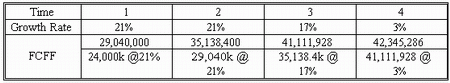### CFA Practice Question

There are 201 practice questions for this study session.

### CFA Practice Question

An analyst is reviewing the following data with respect to York Co.:

• 5 million shares outstanding
• Market risk premium is 6%
• Debt to total capital ratio is 30%
• Risk-free rate is 3%
• York Co beta is 0.92
• Long term debt of \$215 million has an interest cost of 7%
• Tax rate is 40%
• Current free cash flow to the firm (FCFF) is \$24 million
• FCFF is expected to grow 21% over the next two years, 17% in the third year,
• And thereafter, grow at a constant rate of 3%

Which of the following would best estimate the total value of the firm?

A. \$1,008,221,095
B. \$904,210,884
C. \$835,468,669

Step 1. Compute WACC. WACC = 0.3 x 7% x (1 - 0.4) + 0.7 x 8.52% = 7.224%. Note that 8.52% = 3% + 0.92 x 6%.

Step 2: Forecast future period FCFF:Step 3. Compute the terminal value of the firm at the point that its growth rate stabilizes (since growth stabilizes in year 4, we calculate terminal value as of the end of the year 3):

V3 = FCFF4/(WACC - g) = 42,345,286/(0.07224 - 0.03) = 1,002,492,566

Step 4. Compute the PV of all interim FCFFs and the terminal value.

V0 = 29,040,000/1.07224 + 35,138,400/1.072242 + 41,111,928/1.072243 + 1,002,492,566/1.072243 = 904,210,884

User Comment
blackberry1 THAT TAKES LOT OF TIME
aravinda just making sure -- is this right?

debt/capital = debt / (debt + equity)
Allen88 yep, it's right aravinda
REITboy I did it completely differently (wrong, I guess), but got the right answer.

I used Ke of 8.52% instead of WACC to discount back the FCFF (=689), then added back the value of the LT debt.

Don't know why it worked, but it did..
quanttrader wow what a very long section!
endurance pretty straightforward.... 1) WACC, 2) cash flows, 3) term value, 4) CF menu on the BAII
davcer total capital=capital + liabilities
sahilb7 This is too loooooooooooooooooooooong!
ashish100 Just like how my paycheck is going to look like..